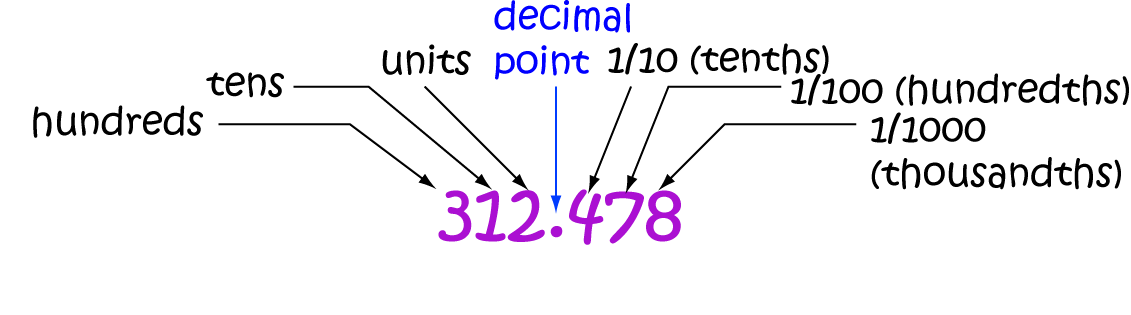# Definition of Place ValuePlace Value is the value of each digit, determined by its position in a numeral.

For example, the $7$ in $387$ is in the "units" position, so it has a value of $7$.

The $8$ in $382$ is in the "tens" position, so it has a value of $80$.

The $5$ in $238.75$ is in the "hundredths" position, so it has a value of $\dfrac{5}{100}$, or $0.05$.

### Description

The aim of this dictionary is to provide definitions to common mathematical terms. Students learn a new math skill every week at school, sometimes just before they start a new skill, if they want to look at what a specific term means, this is where this dictionary will become handy and a go-to guide for a student.

### Audience

Year 1 to Year 12 students

### Learning Objectives

Learn common math terms starting with letter P

Author: Subject Coach
You must be logged in as Student to ask a Question.